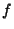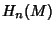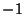## Degree (Map)

Letbe a Map between two compact, connected, oriented-D Manifolds without boundary. Theninduces a Homeomorphismfrom the Homology Groupsto, both canonically isomorphic to the Integers, and socan be thought of as a Homeomorphism of the Integers. The Integerto which the number 1 gets sent is called the degree of the Map.

There is an easy way to computeif the Manifolds involved are smooth. Let, and approximateby a smooth map Homotopic tosuch thatis a regular value'' of(which exist and are everywhere by Sard's Theorem). By the Implicit Function Theorem, each point inhas a Neighborhood such thatrestricted to it is a Diffeomorphism. If the Diffeomorphism is orientation preserving, assign it the number, and if it is orientation reversing, assign it the number. Add up all the numbers for all the points in, and that is the, the degree of. One reason why the degree of a map is important is because it is a Homotopy invariant. A sharper result states that two self-maps of the-sphere are homotopic Iff they have the same degree. This is equivalent to the result that theth Homotopy Group of the-Sphere is the setof Integers. The Isomorphism is given by taking the degree of any representation.

One important application of the degree concept is that homotopy classes of maps from-spheres to-spheres are classified by their degree (there is exactly one homotopy class of maps for every Integer, andis the degree of those maps).